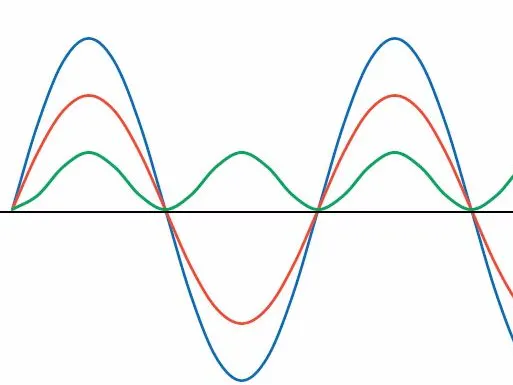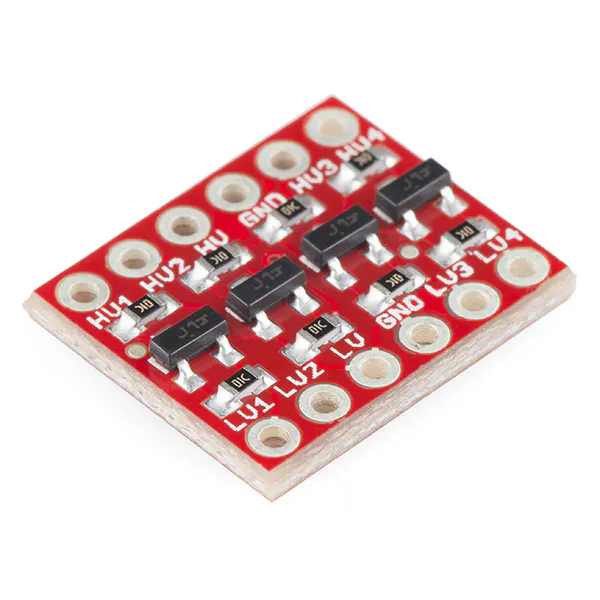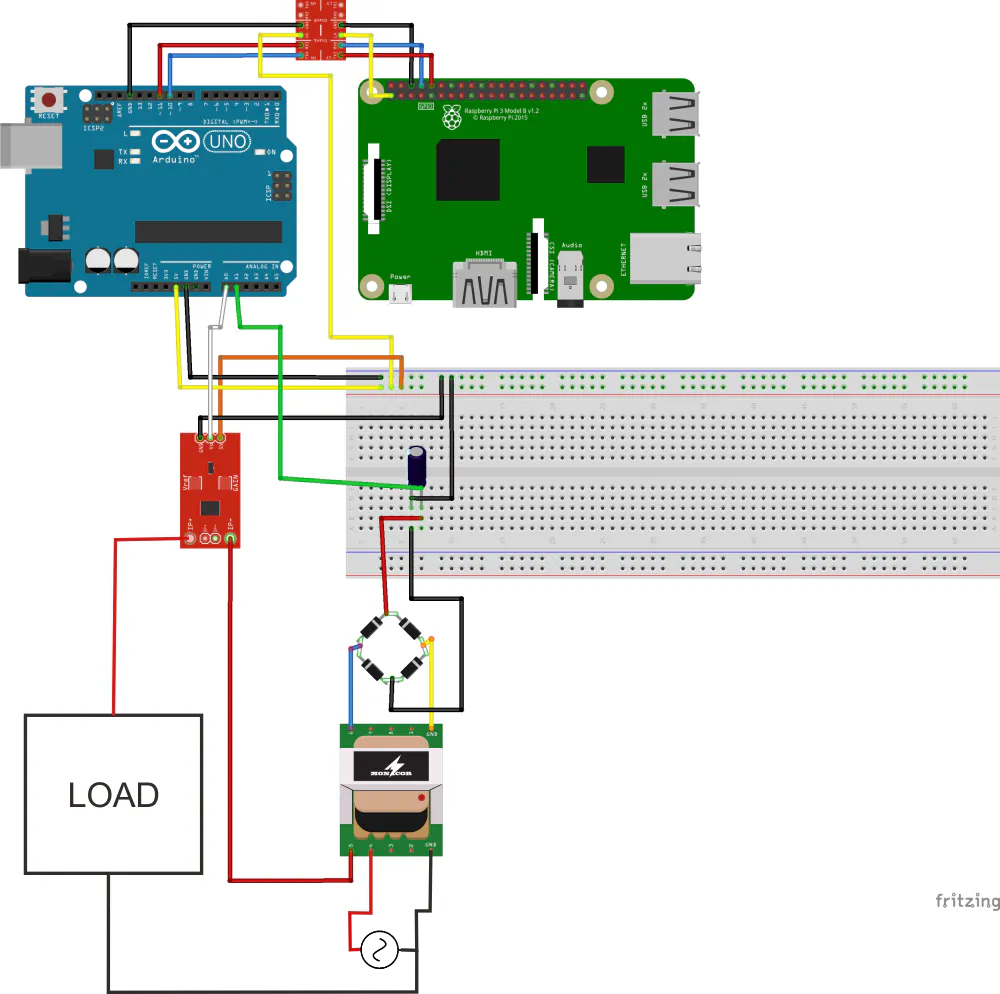Created October 31, 2017

# DEVICE FAULT PREDICTION BASED ON VOLTAGE, CURRENT AND POWER

In industry, there are so many devices that used to manufacture product.If there is a fault we should quickly know where it take place.

54## Things used in this project

### Hardware componentsRaspberry Pi 3 Model B
×1Arduino UNO
×1
 SparkFun ACS712
×1
 Voltage Sensor
×1Jumper wires (generic)
×1×1SparkFun Logic Level Converter - Bi-Directional
×1

### Software apps and online servicesArduino IDEAndroid Studio

## Schematics

### Complete Schematic

Complete hardware schematic## Code

### Arduino Full Code

Arduino
Arduino code to read all sensors and send them to raspberry pi 3
```#include <SoftwareSerial.h>
SoftwareSerial mySerial(10, 11); // RX, TX
int teganganACakhir = 0;
float rms = 0;
float daya = 0;
const int currentPin = A0;
const unsigned long sampleTime = 100000UL;                           // sampling for frequency 50Hz or 60Hz
const unsigned long numSamples = 250UL;                               // sampling divider
const unsigned long sampleInterval = sampleTime/numSamples;  // sampling Interval
void setup() {
// Open serial communications and wait for port to open:
Serial.begin(9600);
while (!Serial) {
; // wait for serial port to connect. Needed for native USB port only
}
mySerial.begin(115200);
}
void loop() { // run over and over
calculatePower(); //call calculate power function
mySerial.print(rms);
mySerial.print("-");
mySerial.print(teganganACakhir);
mySerial.print("-");
mySerial.print(daya);

Serial.print(rms);
Serial.print("-");
Serial.print(teganganACakhir);
Serial.print("-");
Serial.print(daya);
delay(1000);
}
unsigned long currentAcc = 0; //variable currentAcc
unsigned int count = 0; //variable count
unsigned long prevMicros = micros() - sampleInterval ;
while (count < numSamples)
{
if (micros() - prevMicros >= sampleInterval)
{
++count;
prevMicros += sampleInterval;
}
}
rms = sqrt((float)currentAcc/(float)numSamples) * (27.027 / 1024.0); //calculate rms value
rms = rms-0.1; //substract the noise
if (rms<0.04) { //minimize noise
rms=0;
}
}
int i;
for (i=0; i<30; i++) { //read 30 times for data smoothing
delay(2);
}
tegangan = tegangan*(5000/1023.0); //Convert adc value to voltage
float teganganAC = (tegangan/1000)*59.9; //Converting to AC value (about 220V).
teganganACakhir = int(teganganAC); //force to integer
}
void calculatePower() {
daya = rms*teganganACakhir; //power. current multiplied by voltage
}
```

## Credits

### Muh Izzuddin Shofar

0 projects • 1 follower